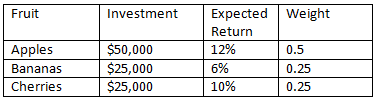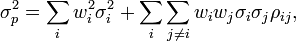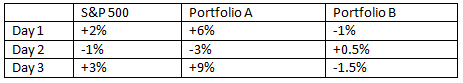## Calculating Expected Portfolio Returns

A portfolio’s expected return is the sum of the weighted average of each asset’s expected return.

### Learning Objectives

Calculate a portfolio’s expected return

### Key Takeaways

#### Key Points

• To calculate the expected return of a portfolio, you need to know the expected return and weight of each asset in a portfolio.
• The figure is found by multiplying each asset’s weight with its expected return, and then adding up all those figures at the end.
• These estimates are based on the assumption that what we have seen in the past is what we can expect in the future, and ignores a structural view on the market.

#### Key Terms

• weighted average: In statistics, a weighted average is an average that takes each object and calculates the product of its weight and its figure and sums all of these products to produce one average. It is implied that all the individual weights add to 1.

Let’s say that we have a portfolio that consists of three assets, and we’ll call them Apples, Bananas, and Cherries. We decided to invest in all three, because the previous chapters on diversification had a profound impact on our investment strategy, and we now understand that diversifiable risk doesn’t pay a risk premium, so we try to eliminate it.A Fruitful Portfolio: How would you calculate the expected return on this portfolio?

The return of our fruit portfolio could be modeled as a sum of the weighted average of each fruit’s expected return. In math, that means:

$\text{E}(\text{R}_{\text{FMP}}) = \text{W}_{\text{A}}(\text{A}*\text{E}(\text{R}_{\text{A}}))+\text{W}_{\text{B}}(\text{B}*\text{E}(\text{R}_{\text{B}}))+\text{W}_{\text{C}}(\text{C}*\text{E}(\text{R}_{\text{C}}))$

Where A stands for apple, B is banana, C is cherry and FMP is farmer’s market portfolio. W is weight and E(RX) is the expected return of X. A good exercise would be to calculate this figure on your own, then look below to see if you completed it accurately.

Here’s what you should get:

$\text{E}(\text{R}_{\text{FMP}})=1.1$

In reality, a portfolio is not a fruit basket, and neither is the formula. A math-heavy formula for calculating the expected return on a portfolio, Q, of n assets would be:

$\text{E}(\text{R}_{\text{Q}}) =\sum_{ \text{i}=1 }^{ \text{n} }{ { \text{w} }_{ \text{i} }\bullet { \text{R} }_{ \text{i} } }$

What does this equal?

$\sum _{ \text{i}=1 }^{ \text{n} }{ { \text{w} }_{ \text{i} } }$

Remember that we are making the assumption that we can accurately measure these outcomes based on what we have seen in the past. If you were playing roulette at a casino, you may not know if red or black (or green) is coming on the next spin, but you could reasonably expect that if you bet on black 4000 times in a row, you’re likely to get paid on about 1900 of those spins. If you go to Wikipedia, you can review a wide variety of challenges to this model that have very valid points. Remember, the market is random: it is not a roulette wheel, but that might be the best thing we have to compare it to.

## Portfolio Risk

The risk in a portfolio is measured as the amount of variance that investors can expect based on historical data.

### Learning Objectives

Calculate a portfolio’s variance

### Key Takeaways

#### Key Points

• Portfolios that are efficient investments are those that effectively diversify the underlying risk away and price their investment efficiently.
• Portfolio risk takes into account the risk and weight of each individual position and also the co- variances across different positions.
• To calculate the risk of a portfolio, you need each asset ‘s variance along with a matrix of cross-asset correlations.

#### Key Terms

• Co-Variance: In probability theory and statistics, co-variance is a measure of how much two random variables change together.
• risk: The potential (conventionally negative) impact of an event, determined by combining the likelihood of the event occurring with the impact, should it occur.
• portfolio: The group of investments and other assets held by an investor.

### Portfolio Risk

An investor can reduce portfolio risk by holding combinations of instruments which are not perfectly positively correlated (correlation coefficient). In other words, investors can reduce their exposure to individual asset risk by holding a diversified portfolio of assets. Diversification may allow for the same portfolio expected return with reduced risk.

Three assets (apples, bananas, and cherries) can be thought of as a bowl of fruit. The index is a a fruit basket. A full fruit basket probably has 10 or 15 different fruits, but my bowl will be efficient as much as its statistical parameters (risk and return) mimic those of the whole basket. In this unit, we are talking about calculating the risk of a portfolio. In addition, we can extend the implications made by the security market line theory from individual assets to portfolios. How does my bowl of fruit compare to the whole basket and how does that compare to other bowls out there?

### Calculating Portfolio Risk

To calculate the risk in my bowl, we need a little more background information on fruit markets. First, we are going to need the variance for each fruit. Remember that the standard deviation answers the question of how far do I expect one individual outcome to deviate from the overall mean. And variance is that number squared. Mathematically, the formula is:

$\text{V}(\text{R})={ [\text{R}-\text{E}(\text{R})] }^{ 2 }$

It is the expected value of the difference between the individual return in a given day (R) and the average outcome average return over a year (E(R)).

In order to calculate the variance of a portfolio of three assets, we need to know that figure for apples, bananas, and cherries, and we also need to know the co-variance of each. Co-variances can be thought of as correlations. If every time bananas have a bad day, so do apples, their co-variance will be large. If bananas do great half of the time when cherries do bad and bananas do terrible the other half, their co-variance is zero. If there is zero correlation among all three fruits, we have cut our risk in thirds by owning all three, but if they are perfectly correlated, we haven’t diversified away any of our risk.

In reality, they are probably positively correlated, since they are all fruits, but not at all perfectly. Apples and bananas grow in different climates so their performance may be a result of weather patterns in either region. Apples may be a substitute for cherries when cherries are expensive. The overall risk of the portfolio would take into account three individual variances and three co-variances (apples-bananas, apples-cherries, and bananas-cherries) and it would reduce the overall portfolio to the degree that they are uncorrelated.

The formula to compute the co-variance between returns on X and Y:

$\text{Cov}(\text{X}|\text{Y})=\text{E}[(\text{X}-\text{E}(\text{X}))(\text{Y}-\text{E}(\text{Y})]$

This means what do I expect to see, in a given time period , when I multiply how much X returned off its average performance from how much Y returned off its average. But notice how it could be positive or negative. And if X tends to be up when Y is down, that would make them two good hedges. And it can be shown that:

From co-variance, we get correlation coefficients:

${ \rho }_{ \text{AB} }=\frac { \text{Cov}(\text{X}|\text{Y}) }{ { \sigma }_{ \text{A} }^{ 2 }\cdot { \sigma }_{ \text{B} }^{ 2 } }$

In finance and statistics, the greek letter rho squared represents variance.

So now that we have figures to help us measure the risk and reward of our individual fruit bowl, we can go look at historical figures to determine the expected returns and the risk of the index that comprises the entire fruit basket industry. If our portfolio of investments has diversified away as much risk as is possible given the costs of diversifying, our portfolio will be attractive to investors. If our bowl does not diversify away enough risk, it will not lie on the Security Market Line for those who we are trying to recruit into buying our portfolio.

The same principles that were applied to individual investments in the Understanding the SML section can be applied to the market for portfolio investments. If an institutional investor, such as a city pension fund, looked at two portfolios with identical returns and different risks, they would choose the portfolio that minimized its risk. Thus the only portfolios that are efficient investments are those that effectively diversify the underlying risk away and price their investment efficiently.Variance of any portfolio: The formula shows that the overall variance in a portfolio is the sum of each individual variance along with the cross-asset correlations.

## Beta Coefficient for Portfolios

A portfolio’s Beta is the volatility correlated to an underlying index.

### Learning Objectives

Calculate a portfolio’s beta

### Key Takeaways

#### Key Points

• In individual stocks, a beta coefficient compares how much a particular stock fluctuates in value on a day-to-day basis.
• A beta coefficient for a portfolio of assets measures how that portfolio value changes compared to a benchmark, like the S&P 500. A value of 1 suggests that it fluctuates as much as the index and in the same direction.
• A beta coefficient of less than 1 suggests a portfolio that fluctuates less than the benchmark. A negative beta is an indication that a portfolio moves in the opposite direction of its benchmark.

#### Key Terms

• Normalized variable: In statistics, a normalized variable is one that is calculated using a ratio of itself and some benchmark figure. Normalized figures tend to be smaller than the original values.

In this section, we will discuss the idea of calculating a Beta coefficient to help investors measure the risk -reward trade-off for a blended pool of investments.

In this case, it is important to remember that a portfolio may represent the seller side of the market and the buyer can be thought of an institutional investor or a mutual fund. A portfolio’s Beta is the volatility correlated to an underlying index. If we think of the S&P 500 as the index, a portfolio that fluctuates identically to the market has a Beta of 1. What would the following portfolios have for Beta values?Calculating Beta: Two hypothetical portfolios; what do you think each Beta value is?

Do you have an answer? Beta is a normalized variable, which means that it is a ratio of two variances, so you have to compare the volatility of returns to the benchmark volatility. Portfolio A has a direct relationship with the S&P 500 – it is scaled by three times each day. When the market is up 2%, it is up 6%. Thus, the portfolio would have a Beta value of 3. Portfolio B is a different situation; it is also directly proportional, but in the negative direction. Every time the market is up 1%, the portfolio is down half a percent. The Beta for this portfolio, when compared with the S&P 500 benchmark, would be -0.5. A Beta of zero in this situation doesn’t necessarily mean a risk free asset, it simply means that it is not correlated with the benchmark.

In reality, the numbers would rarely work out this cleanly, but this is a good model to demonstrate some key concepts. A pension fund that seeks to maximize its reward and limit its risk might be interested in each of these portfolios. If you invested equal amounts in each portfolio, it would leave you over-exposed to the market because it would have a Beta of 1.5. But let’s say you have \$300,000 to invest; you could put that in a fund that is indexed to the S&P 500 and is perfectly correlated with it.

Every time the S&P gains 1%, your fund nets you 100,000 in fund A and S Misplaced &3,000 and your position in fund B pays you 2,000, which is less damage than you would have suffered on your position in the S&P index fund. On days when the S 3,000 and your fund A position loses 2,000 and your upside is limited by the same amount, your downside is reduced.

A pension fund is a good example of an institutional client that could extend the principles of diversification to a pool of blended portfolios. A city with an aging workforce needs to be protected from downside risk. It is the same principle as an employee approaching retirement; it can afford to have a heavier position in the S&P 500 if it has a position in portfolio B.